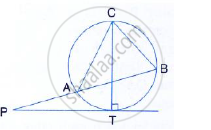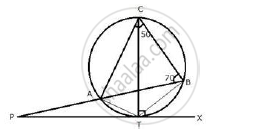Share

Pt is a Tangent to the Circle at T. If ∠Abc = 70° and ∠Acb = 50°; Calculate: (I) ∠Cbt (Ii) ∠Bat (Iii) ∠Apt - ICSE Class 10 - Mathematics

ConceptTangent Properties - If a Line Touches a Circle and from the Point of Contact, a Chord is Drawn, the Angles Between the Tangent and the Chord Are Respectively Equal to the Angles in the Corresponding Alternate Segments

Question

PT is a tangent to the circle at T. if ∠ABC = 70° and ∠ACB = 50°; Calculate:
(i) ∠CBT (ii) ∠BAT (iii) ∠APTSolution 1Join AT and BT.
i) TC is the diameter of the circle
∴ ∠CBT =  90°  (Angle in a semi – circle)
(ii) ∠ CBA =  70°
∴ ∠ABT = ∠CBT - ∠CBA = 90°  - 70 °  = 20°
Now, ∠ ACT  = ∠ABT =  20°  (Angle in the same segment of the circle)

∴∠ TCB = ∠ACB - ∠ACT =  50° - 20° = 30°
But, ∠ TCB  = ∠TAB (Angles in the same segment of the circle)
∴ ∠ TAB or ∠ BAT = 30°
(iii) ∠BTX  = ∠TCB = 30 °(Angles in the same segment)
∴ ∠ PTB = 180° - 30° =150°

Now in Δ PTB
∠ APT  + ∠PTB  +∠ ABT = 180°
⇒ ∠APT + 150 ° + 20° =  180°
⇒ ∠ APT =  180° -  170° = 10°

Solution 2Join AT and BT.
i) TC is the diameter of the circle
∴ ∠CBT =  90°  (Angle in a semi – circle)
(ii) ∠ CBA =  70°
∴ ∠ABT = ∠CBT - ∠CBA = 90°  - 70 °  = 20°
Now, ∠ ACT  = ∠ABT =  20°  (Angle in the same segment of the circle)

∴∠ TCB = ∠ACB - ∠ACT =  50° - 20° = 30°
But, ∠ TCB  = ∠TAB (Angles in the same segment of the circle)
∴ ∠ TAB or ∠ BAT = 30°
(iii) ∠BTX  = ∠TCB = 30 °(Angles in the same segment)
∴ ∠ PTB = 180° - 30° =150°

Now in Δ PTB
∠ APT  + ∠PTB  +∠ ABT = 180°
⇒ ∠APT + 150 ° + 20° =  180°
⇒ ∠ APT =  180° -  170° = 10°

Is there an error in this question or solution?

Video TutorialsVIEW ALL 

Solution Pt is a Tangent to the Circle at T. If ∠Abc = 70° and ∠Acb = 50°; Calculate: (I) ∠Cbt (Ii) ∠Bat (Iii) ∠Apt Concept: Tangent Properties - If a Line Touches a Circle and from the Point of Contact, a Chord is Drawn, the Angles Between the Tangent and the Chord Are Respectively Equal to the Angles in the Corresponding Alternate Segments.
S